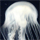## General Question# How many possible positive and negative zeros are in 3x^5 + 6x?

Asked by mathhelp1221 (11) October 14th, 2014 from iPhone

I have a question about Descartes’ rule of signs, and I can’t figure out how many possible positive and negative zeros there are in 3x^5 + 6x. Can somebody help? Thanks!

Observing members: 0Composing members: 0You do not need the rule of signs to determine the number of positive and negative roots. Start by factoring out x to get x(3x^4 + 6) = 0. We have one root at x=0. Let’s check for the solutions of 3x^4 + 6 = 0. Rearranging the terms gives x^4 = -2. This has no real solutions, positive or negative, since any real number raised to an even power must be greater than or equal to 0.

LostInParadise (27572)“Great Answer” (6) Flag as…To use the sign rule, notice that the two coefficients have the same sign (3 and 6 are both positive), so there is no sign change in f(x), which means that there are no positive real roots. To work with the negative real roots, find f(-x) = -3x^5 – 6x. Again there are no sign changes, so there are no negative real roots.

LostInParadise (27572)“Great Answer” (2) Flag as…or